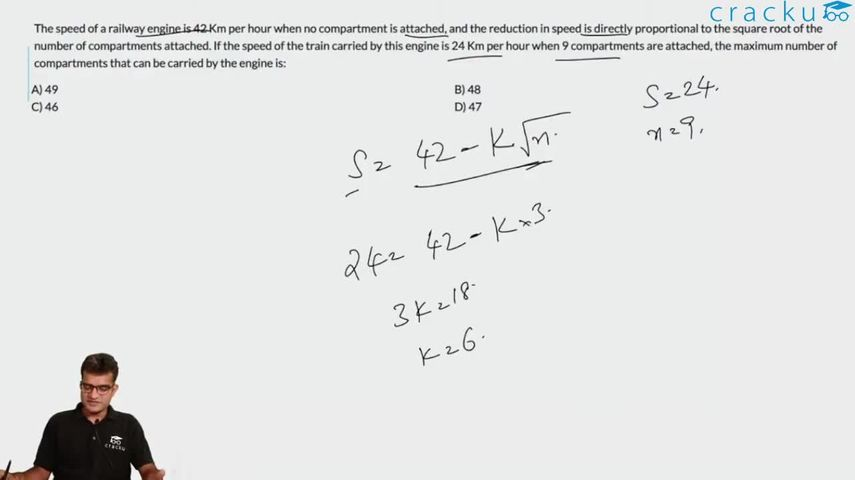Question 6

# The speed of a railway engine is 42 Km per hour when no compartment is attached, and the reduction in speed is directly proportional to the square root of the number of compartments attached. If the speed of the train carried by this engine is 24 Km per hour when 9 compartments are attached, the maximum number of compartments that can be carried by the engine is:

Solution

The function of the speed of the train = 42 - k$$\sqrt{n}$$ where n is the number of compartments and k is a constant.

42 - k$$\sqrt{9}$$ = 24

=> 3k = 18 => k = 6

=> Function of speed = 42 - 6$$\sqrt{n}$$

Speed is 0 when 42 - 6$$\sqrt{n}$$ = 0

=> 42 = 6$$\sqrt{n}$$

=> n = 49

=> So, with a positive speed, the train can carry 48 compartments.

### View Video Solution